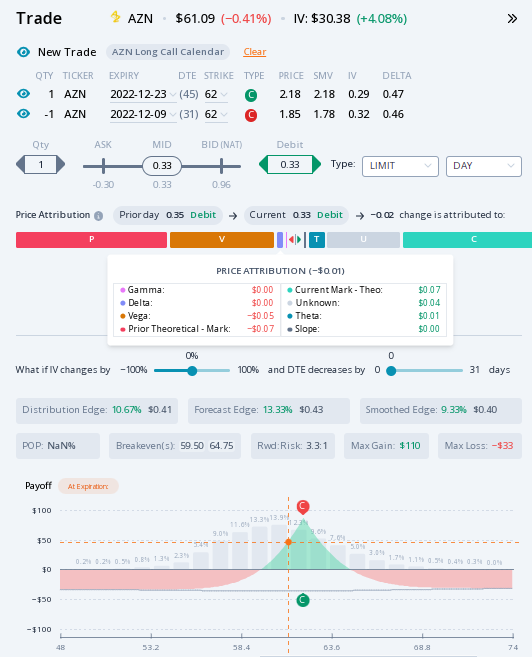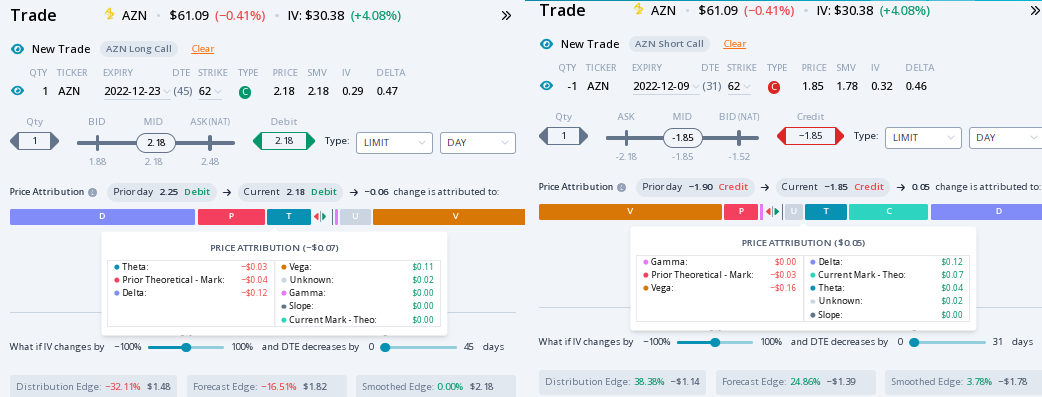The profit attribution works for existing positions and for trades you are considering.

For example, lets say you are considering a calendar spread in Astra Zeneca (AZR) before earnings tomorrow. The December 23rd, 9th \$62 strike call spread is priced at \$0.33 now down from \$0.35 yesterday. What is that \$0.02 difference attributed?As shown in the Price Attribution (not called profit attribution because you do not have a position), we attribute that difference to the following factors: Delta, Gamma, Vega, Prior and Current Marks, Theta, Slope, and Unknown.

The delta of a call will add to the profit if the stock is up. The gamma will add to any long option where the stock moved. The vega will add to the profit of a long option if the IV is up. The theta will add to profit for any short options. The current mark minus the theoretical value will add to profit if greater than zero. The prior theoretical value minus the prior mark will add to the profit if greater than zero. The put-call slope will add to the profit for long put options. Unknown is what's left over after adding all these attributions together and comparing to the actual change.

For this spread, you can add the profit attributions of each call together to find the total.The delta offset. The gamma was zero for both. The vega interestingly was net negative with the IV up. This happened because the IV was up more for the short call than the long call. The theta was greater for the short-term option. The current mark minus the theoretical value added. The prior theoretical value minus the prior mark subtracted from the total. The put-call slope change had no effect. Unknown was additive.

Give this feature a try at https://dashboard.orats.com